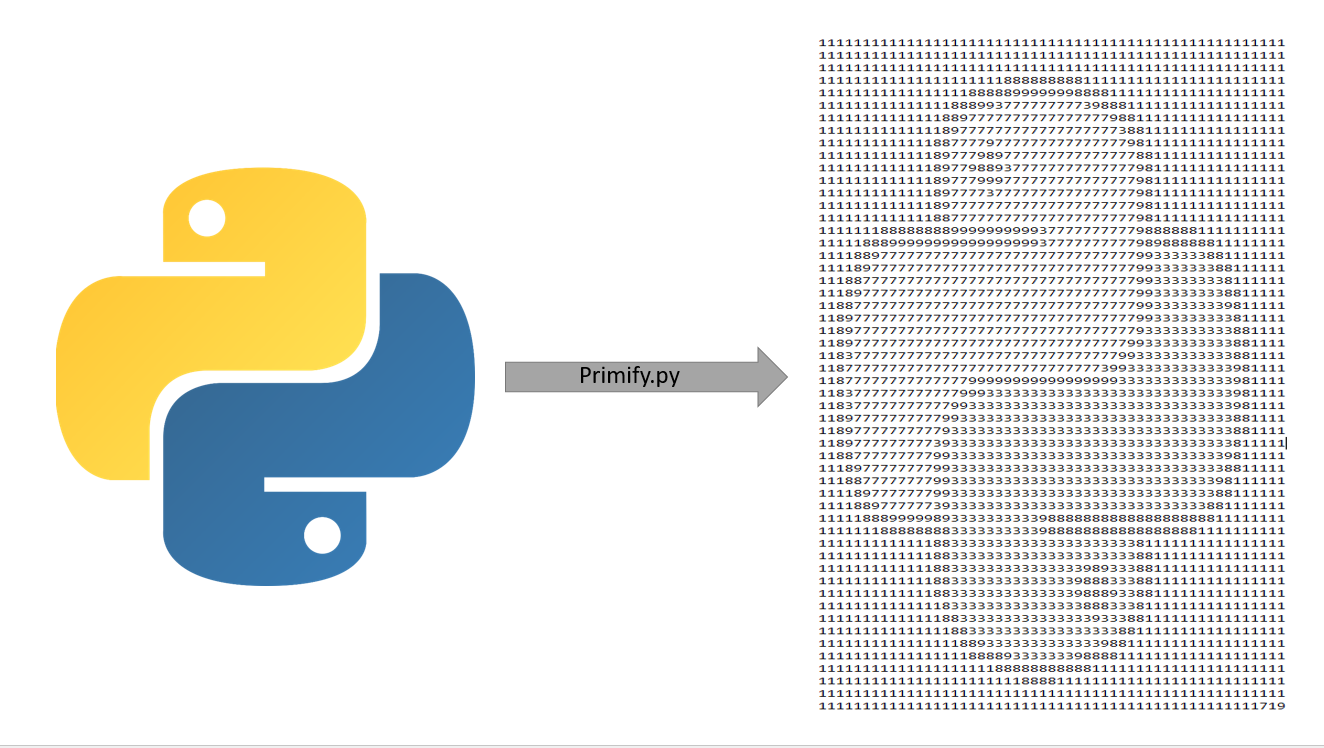Embed any image into a prime number.

# Primify

Transform any image into a prime number that looks like the image if glanced upon from far away.## How does it work

We proceed in 5 steps:

1. We resize the image to contain at most a --max-digits amount of pixels.

2. Run various image processing steps like edge enhancement and smoothing before converting the image into grey-scale.

3. We then quantise the image into just having 5 to 10 greyness levels.

4. Now we map each greyness level to a digit, et voila, we have embedded the picture into a number.

5. It now remains to tweak some of the digits until we find a prime number that still looks like the image.

Note: According to the prime number theorem, the density of prime numbers is asymptotically of order 1/log(n). Hence, if we have some number n with m digits, the number of primality tests that we expect to do until we hit a prime number is roughly proportional to m. Since we use the Baillie–PSW primality test, the overall expected computational complexity of our prime searching procedure is O(n*log(n)³).

## How to use

Simply get the primify command line tool via pip install primify. You can also import the PrimeImage class from primify.base or use cli.py as a command-line script.

### Command-line tool

usage: primify [-h] [--image IMAGE_PATH] [--max-digits MAX_DIGITS]
[--output-file OUTPUT_FILE]

Command-line tool for converting images to primes

optional arguments:
-h, --help            show this help message and exit
--image IMAGE_PATH, -i IMAGE_PATH
Source image to be converted
--max-digits MAX_DIGITS, -d MAX_DIGITS
Maximal number of digits the prime can have
--output-file OUTPUT_FILE, -o OUTPUT_FILE
File name of the file containing the prime.



Thus, if you have the source image at ./source.png and you want to convert it into a prime contained in ./prime.txt which has at most 5000 digits. Then you should run:

primify -v --image ./source.png --max-digits 5000 --output-file prime.txt

### Importing the PrimeImage class

You can also simply import the PrimeImage class from primify.base and use that class in your own code. Check the documentation for details on how to interact with the underlying API.

Daniel Temkin wrote a lovely article on his blog esoteric.codes giving some interesting insight and background for this tool. You can read it here.

## Project details

This version2.0.01.0.21.0.1

Uploaded py2 py3